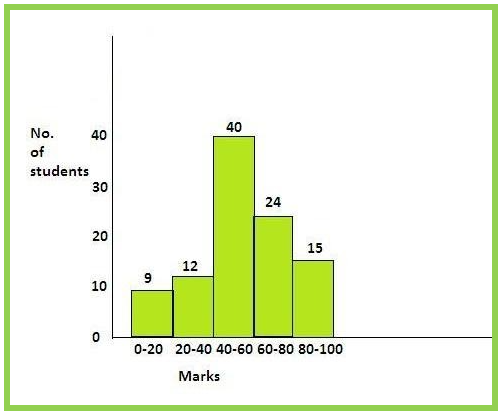QuestionAnswers

# The following is the frequency distribution of marks:$\begin{array}{*{20}{c}} {Marks}&{0 - 20}&{20 - 40}&{40 - 60}&{60 - 80}&{80 - 100}&{Total} \\ {Frequency}&9&a&{40}&b&{15}&{100} \end{array}$The value of b is twice the value of a. Find their values and draw their histogram.

Hint: We will make two equations for two unknown variables a and b. First by using the information that the value of b is twice the value of a and secondly the total of all the frequencies is 100.
We will eventually solve these both equations to get the required values and put the values in the table and then create a histogram.

We are provided in the question that:- the value of b is twice the value of a.
This statement is equivalent to b = 2$\times$a
So, we have:- b = 2a ……(1)
Now, we also know that there are a total of 100 students. So, the sum of all the students in each category of marks will result in 100.
Hence, 9 + a + 40 + b + 15 = 100
Clubbing all the numeric values on L.H.S. to simplify the expression, we get:-
a + b + (9 + 40 + 15) = 100
Simplifying the expression, we see that:-
a + b + 64 = 100
Taking 64 to R.H.S, we get:-
a + b = 100 – 64
Simplifying it further, we will get:-
a + b = 36 …….(2)
Now, we have got two equations (1) and (2) with 2 unknown variables a and b.
Putting (1) in (2), we will have:-
a + 2a = 36
Simplifying the L.H.S, we will get:-
3a = 36
Taking 3 on R.H.S, we will get:-
a = $\dfrac{{36}}{3} = 12$
Hence, we get a = 12.
Putting this value of a in (1),
b = 2a = 2$\times$12 = 24
Thus, we have a = 12 and b = 24.
Now, let us draw the histogram based on the given data and the found values:Note: Always remember the fact that we need as much equations, as much unknown variables we have in our question. Here, we had a and b, so we formed two equations using the data provided to us. If there would have been more than 2 variables, we would already be provided with some more information in the question only to form more equations.